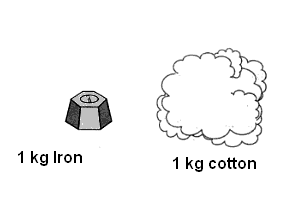Determination of Density of Solid

Our Objective

To determine the density of a solid (which is denser than water) by using a spring balance and a measuring cylinder.

The Theory

All matter has mass and volume. Mass and volume are the  physical properties of matter and may vary with different objects The amount of matter contained in an object is called mass. Its measure is usually given in grams (g) or kilograms (kg). Volume is the amount of space occupied by an object. The units for volume including liters (l), meters cubed (m3), and gallons (gal).

Consider two different substances such as iron and cotton of same mass. It is observed that Iron will occupy less volume as compared to cotton. This is due to their differences in density. Density of Iron is more than that of cotton.The mass of a unit volume of a substance is called its density.If D is the density of a body of mass M and volume V, thenIn S.I units density is expressed in kg m -3.

For example,  density of water is 1000 kg m-3.

Density of iron is 8500 kg m-3.

Most of the substances expand on heating and contract on cooling, but the mass remaining constant for all cases. The density of most of the substances decreases with the increase in temperature and increases with decrease in temperature. But water contracts when cooled up to 40C but expands when cooled further below 40C . Thus the density of water is maximum at 40C.

Relative density of a substance is defined as the ratio between the density of the substance to the density of water at 40C. Relative density is also known as specific gravity. The relative density of a substance is a pure number without any unit. It tells how many times a substance is heavier than water.

Relative density (R.D) of a substance can be calculated by dividing density of a substance with the density of water.For example,Thus, Relative density of iron is 8.5 (No unit is applied).

Density of a substance can be calculated by multiplying Relative Density (R.D.) of the substance with the Density of water.Spring Balance:  It is a device used for the determination of gravitational mass of a body. It works on the principle of Hooks law of elasticity which states that when a body is suspended from a vertical spring, the body produces extension in the length of the spring proportional to its gravitational mass.

Learning outcomes

1. The student will understand the concepts of mass, volume and density of an object.
2. The student can calculate density of a solid heavier than water by measuring its mass and volume.

Cite this Simulator: